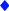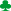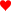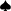# How To Work Out Hand Probability In Texas HoldemEver wondered where some of those odds in the odds charts came from? In this article, I will teach you how to work out the probability of being dealt different types of preflop hands in Texas Holdem.

It's all pretty simple and you don't need to be a mathematician to work out the probabilities. I'll keep the math part as straightforward as I can to help keep this an easy-going article for the both of us.

## A few probability basics.

When working out hand probabilities, the main probabilities we will work with are the number of cards in the deck and the number of cards we want to be dealt. So for example, if we were going to deal out 1 card:

• The probability of dealing a 7would be 1/52 - There is one 7in a deck of 52 cards.
• The probability of dealing any Ace would be 4/52 - There four Aces in a deck of 52 cards.
• The probability of dealing anywould be 13/52 - There are 13s in a deck of 52 cards.

In fact, the probability of being dealt any random card (not just the 7) would be 1/52. This also applies to the probability being dealt any random value of card like Kings, tens, fours, whatever (4/52) and the probability of being dealt any random suit (13/52).

Each card is just as likely to be dealt as any other - no special priorities in this game!

### The numbers change for future cards.

A quick example... let's say we want to work out the probability of being dealt a pair of sevens.

• The probability of being dealt a 7 for the first card will be 4/52.
• The probability of being dealt a 7 for the second card will be 3/51.

Notice how the probability changes for the second card? After we have been dealt the first card, there is now 1 less card in the deck making it 51 cards in total. Also, after already being dealt a 7, there are now only three 7s left in the deck.

Always try and take care with the numbers for future cards. The numbers will change slightly as you go along.

### Working out probabilities.

• Whenever the word "and" is used, it will usually mean multiply.
• Whenever the word "or" is used, it will usually mean add.

This won't make much sense for now, but it will make a lot of sense a little further on in the article. Trust me.

## Probability of being dealt two exact cards.

Multiply the two probabilities together.

So, we want to find the probability of being dealt the Aand K. (See the "and" there?)

• Probability of being dealt A- 1/52.
• Probability of being dealt K- 1/51.

Now let's just multiply these bad boys together.

P = (1/52) * (1/51)
P = 1/2652

So the probability of being dealt the Aand then Kis 1/2652. As you might be able to work out, this is the same probability for any two exact cards, as the likelihood of being dealt AKis the same as being dealt a hand like 73in that order.

### But wait, we do not care about the order of the cards we are dealt!

When we are dealt a hand in Texas Hold'em, we don't care whether we get the Afirst or the Kfirst (which is what we just worked out), just as long as we get them in our hand it's all the same. There are two possible combinations of being dealt this hand (AKand KA), so we simply multiply the probability by 2 to get a more useful probability.

P = 1/2652 * 2
P = 1/1326

You might notice that because of this, we have also worked out that there are 1,326 possible combinations of starting hands in Texas Holdem. Cool huh?

## Probability of being dealt a certain hand.

Two exact cards is all well and good, but what if we want to work out the chances of being dealt AK, regardless of specific suits and whatnot? Well, we just do the same again...

Multiply the two probabilities together.

So, we want to find the probability of being dealt any Ace and any King.

• Probability of being dealt any Ace - 4/52.
• Probability of being dealt any King - 4/51 (after we've been dealt our Ace, there are now 51 cards left).

P = (4/52) * (4/51)
P = 16/2652 = 1/166

However, again with the 2652 number we are working out the probability of being deal an Ace and then a King. If we want the probability of being dealt either in any order, there are two possible ways to make this AK combination so we multiply the probability by 2.

P = 16/2652 * 2
P = 32/2652
P = 1/83

The probability of being dealt any AK as opposed to an AK with exact suits is more probable as we would expect. A lot more probable in fact. Also, as you might guess, this probability of 1/83 will be the same for any two value of cards like; AQ, JT, 34, J2 and so on regardless of whether they are suited or not.

## Probability of being dealt a range of hands.

Work out each individual hand probability and add them together.

What's the probability of being dealt AA or KK? (Spot the "or" there? - Time to add.)

• Probability of being dealt AA - 1/221 (4/52 * 3/51 = 1/221).
• Probability of being dealt KK - 1/221 (4/52 * 3/51 = 1/221).

P = (1/221) + (1/221)
P = 2/221 = 1/110

Easy enough. If you want to add more possible hands in to the range, just work out their individual probability and add them in. So if we wanted to work out the odds of being dealt AA, KK or 73...

• Probability of being dealt AA - 1/221 (4/52 * 3/51 = 1/221).
• Probability of being dealt KK - 1/221 (4/52 * 3/51 = 1/221).
• Probability of being dealt 73- 1/1326 ([1/52 * 1/51] * 2 = 1/1326).

P = (1/221) + (1/221) + (1/1326)
P = 359/36465 = 1/102

This one definitely takes more skill with adding fractions because of the different denominators, but you get the idea. I'm just teaching hand probabilities here, so I'm not going to go in to adding fractions in this article for now! This fractions calculator is really handy for adding those trickier probabilities quickly though.

## Overview of working out hand probabilities.

Hopefully that's enough information and examples to allow you to go off and work out the probabilities of being dealt various hands and ranges of hands before the flop in Texas Holdem. The best way to learn how to work out probabilities is to actually try and work it out for yourself, otherwise the maths part will just go in one ear and out the other.

I'm sure that some of you reading this article were not aware that the probability of being dealt AA were exactly the same as the probability of being dealt 22! Well, now you know - it's 1/221.

### Other useful articles.

Go back to the poker odds charts.

Have You Not Heard Of
Deuces Cracked?“I quit my job and started playing poker back in February of 08',
after a month of losing over 50BI at micro stakes, I joined DC. I've
been living off poker ever since; if anything in this world could help
you become a winning player, this is it.”
- Malefiicus, DC Member

# Subscribe to thepokerbank

I'll send you an email if I add something new and interesting to the website.

Don't worry, it doesn't happen very often.